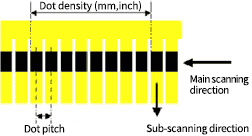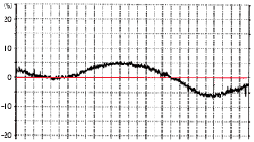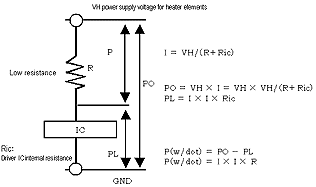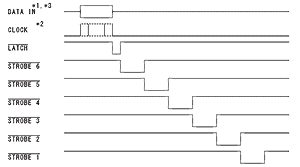Printing Devices

# Technical Terms

## Dot Density

The density of heater elements. It is shown either by [dot/mm] which means the number of heater elements in 1mm, or by [dot/inch = dpi] which means the number of heater elements in 1inch.

## Dot Pitch

Is the inverse number of dot density. Dot pitch is the distance between adjacent heater elements. For example, dot pitch of a 8dot/mm printhead is 0.125mm by 1/8mm.## Heater Size

Each heater size is shown by W x L: Width in main-scanning direction x Length in sub-scanning direction.
In case of a typical 8dot/mm printhead, its heater size is shown as 0.125mm x 0.152mm.

## Resistance Value(=R-value)* Kyocera Internal data
Average resistance value (Rav) Average of resistance value of all heater elements of a printhead (shown by the red line). Maximum deviation of resistance value within a printhead on both the +side and the -side from the Rav of the printhead. It is usually shown as +/-5%. Maximum difference of resistance value between two adjacent heater elements.Kyocera is able to adjust the value to less than +/-1% when required.

## Applied EnergyElectric current per any one dot (I) can be calculated using the above formula, when R is the resistance value of the heater element and Ric is the driver IC's internal resistance.
Applied power is then calculated as above and is shown in (w/dot).
When one pulse time is shown as Ton(msec), applied energy (mJ/dot) can be calculated by the formula, P X Ton.

## Driving Method

DATA（IN） On/Off data for all heater elements. A timing pulse to trigger the DATA transfer into the shift-register in driver IC. A timing pulse to forward DATA in the shift-register into output stage. ON time is controlled by STROBE time.

### TIMING DIAGRAM*A sequence of On/Off DATA is transferred by CLOCK (triggered by the signal edge upon start), and then is stored in the shift-register. The transfer speed is determined by the CLOCK frequency, which is 16MHz maximum depending on the driver IC.
DATA stored in the shift-register is transferred to the output stage by the LATCH signal (negative logic).
Only while STROBE signal (negative logic) is On (at GND voltage), heater elements are heated using the current flow.
(Above Timing Diagram shows an example that makes 1 line print with 6 split strobes)

## Number of simultaneous printable dots

The number of simultaneous printable dots is 8 dots / mm and for A4 size (216 mm), there are 1,728 dots in all.
When 8 mA of current flows in each dot, if all the dots are made at the same time, a current of 13.824 A flows and a large capacity power supply is required.
Therefore, by dividing one line into a plurality of blocks and causally conferring on each block, it becomes possible to use a power supply with a smaller capacity
Although it is divided into 6 in the above figure, in this case 2.304 A is the maximum flowing current.
Since the wiring resistance will cause loss due to the current amount even in the thermal printhead, the number of dots that can be printed simultaneously is set taking this therapy into consideration.

## Thermistor

The temperature of a printhead rises as it continues to print, and the temperature has negative effects on the driver IC.
Moreover the heat accumulation in the printhead has a negative influence on the print quality. A thermistor to monitor the ambient temperature and the printhead temperature, is mounted on the printhead. A thermistor has a characteristic; the higher the temperature, the lower the resistance value from a reference resistance value at 25 degree C. Using this characteristic, temperature can be obtained by the following formula:

T = 1/(2.303/B x Log(R/R25)+1/298)-273

B constant: a coefficient of the thermistor itself resistance value at a temperature a reference resistance value at 25 degree C